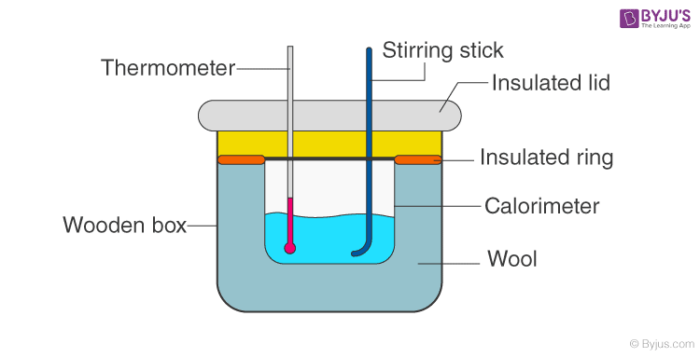Checkout JEE MAINS 2022 Question Paper Analysis : Checkout JEE MAINS 2022 Question Paper Analysis :

# Enthalpy of Neutralization of Strong Acid and Strong Base

Enthalpy of neutralization is always constant for a strong acid and a strong base: this is because all strong acids and strong bases are completely ionized in dilute solution. Enthalpy changes in neutralization are always negative-when an acid and alkali react, heat is given out.

## Aim:

To determine the enthalpy of neutralization of strong acid (hydrochloric acid) and strong base (sodium hydroxide).

## Theory:

Heat is evolved during neutralization of an acid with alkali. The neutralization reaction of a strong acid with a strong base is essentially the combination of one equivalent of hydrogen ions with one equivalent of hydroxyl ions.

Enthalpy of neutralization is the heat evolved when one gram equivalent of the acid is completely neutralized by a base in dilute solution.

The chemical reaction is given below.

H+ + OH → H2O + 13.7 kcal

H+(aq) + Cl(aq) + Na+(aq) + OH(aq) → Na+(aq) + Cl(aq) + H2O + 13.7 kcal

13.7 kcal of heat is liberated out and is the heat of neutralization for all strong acids and bases. Hess in 1840 obtained a constant value of 13.7kcal as the heat of neutralization in almost all the cases of strong acids and strong bases. This constancy of heat of neutralization of a strong acid and strong bases is explained on the basis of ionic theory.

## Materials Required:

1. Wide polythene bottle
2. Rubber cork
3. Thermometer
4. Stirrer fitted with a cork
5. Measuring cylinder
6. 0.2M Hydrochloric acid
7. 0.2M Sodium hydroxide solution

## Apparatus Setup:## Procedure:

1. Calculate the water equivalent of the calorimeter.
2. Place 100ml of 0.2M hydrochloric acid solution in it.
3. Now record the temperature of the acid solution.
4. Take another separate vessel and place 100ml of 0.2M sodium hydroxide solution in it.
5. Note down the initial temperature of sodium hydroxide taken.
6. Wait till both the solution attains the same temperature.
7. Now transfer 100ml of sodium hydroxide solution into the hydrochloric acid quickly.
8. Fit the cork immediately which has a thermometer and a stirrer in the mouth.
9. Stir well the solution and note down the temperature after small intervals of time.
10. Keep noting down the temperature till the temperature becomes constant.
11. Record the highest temperature reached.
12. Calculate the heat evolved when the two solutions are mixed by ratio proportion method.

## Observation and Inference:

 Initial temperature of the acid and base t1oC Final temperature after neutralization t2oC Change in temperature Δt (t1-t2)oC Mass of the mixture solution after neutralization 200g Water equivalent of calorimeter W g

## Calculations:

Enthalpy change during neutralization of 100ml of 0.2M HCl

=

$$\begin{array}{l} (200 \times W) \times (t_{1}-t_{2}) \times 4.2\end{array}$$

Therefore, the enthalpy change during neutralization of 1000ml of 1M HCl

=

$$\begin{array}{l}\frac{(200 \times W) \times (t_{1}-t_{2}) \times 4.2 }{0.2} \times \frac{1000}{100}\end{array}$$
 Enthalpy of neutralization = $$\begin{array}{l}\frac{(200 \times W) \times (t_{1}-t_{2}) \times 4.2 }{0.2\times 100}\end{array}$$kJ

## Results and Discussions:

The enthalpy of neutralization of HCl with NaOH is ________ kJ.

## Precautions:

1. Due to radiation, some heat is lost to the environment.
2. The solution density is supposed to be 1g / ml.
3. Hydrochloric acid and sodium hydroxide ionization is assumed to be 100 %.
4. The solution’s specific heat is taken as 4.189J/g
5. The mixture of HCl and NaOH should be stirred well.
6. Water equivalent calorimeter or beaker should be calculated accurately.

## Frequently Asked Questions on Enthalpy of Neutralization of Strong Acid and Strong Base

### Define the enthalpy of solution.

Enthalpy of solution or solution heat is expressed in kJ / mol, and when a solution is formed it is the amount of heat energy that is released or absorbed.

### Find out whether neutralization reaction is exothermic or endothermic reaction?

It examines the neutralization of a strong acid with a strong base and finds that it always adds heat to the environment. It is important to understand that bonds are made and energy is released into the environment during an exothermic reaction. So neutralization reaction is exothermic in nature.

### What is meant by exothermic and endothermic reactions ?

An exothermic reaction is known as a chemical reaction in which heat is released. An endothermic reaction is called a chemical reaction in which heat energy is absorbed.

### Why is potassium nitrate used in powdered form?

Potassium nitrate used in powdered form because the dissolution will be faster in powdered form.

### What is meant by water equivalent of the calorimeter?

A calorimeter’s water equivalent is nothing but the amount of water that absorbs the same amount of heat that is absorbed by the calorimeter.

Test your knowledge on Enthalpy Of Neutralization Of Strong Acid And Strong Base